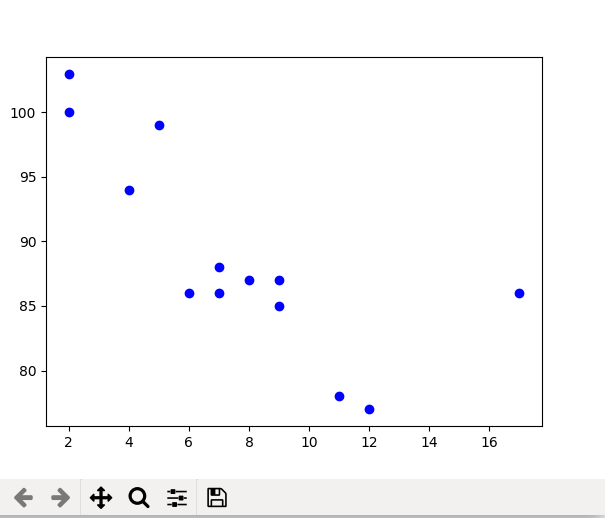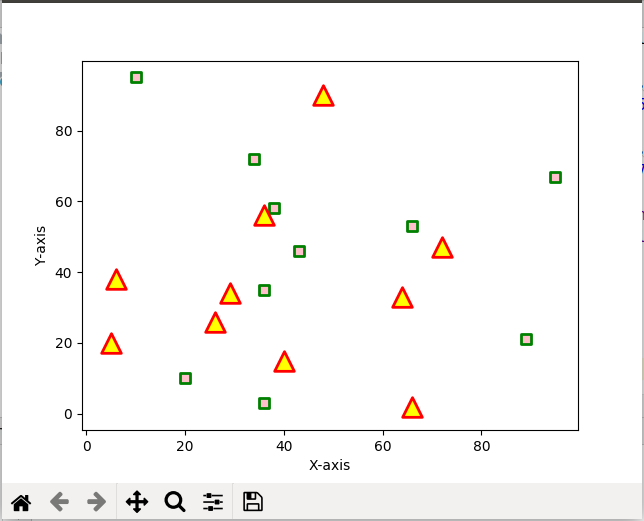Related Articles

# matplotlib.pyplot.scatter() in Python

• Last Updated : 21 Apr, 2020

Matplotlib is a comprehensive library for creating static, animated, and interactive visualizations in Python. It is used for plotting various plots in Python like scatter plot, bar charts, pie charts, line plots, histograms, 3-D plots and many more. We will learn about the scatter plot from the matplotlib library.

## matplotlib.pyplot.scatter()

Scatter plots are used to observe relationship between variables and uses dots to represent the relationship between them. The scatter() method in the matplotlib library is used to draw a scatter plot. Scatter plots are widely used to represent relation among variables and how change in one affects the other.
Syntax
The syntax for scatter() method is given below:

matplotlib.pyplot.scatter(x_axis_data, y_axis_data, s=None, c=None, marker=None, cmap=None, vmin=None, vmax=None, alpha=None, linewidths=None, edgecolors=None)

The scatter() method takes in the following parameters:

• x_axis_data- An array containing x-axis data
• y_axis_data- An array containing y-axis data
• s- marker size (can be scalar or array of size equal to size of x or y)
• c- color of sequence of colors for markers
• marker- marker style
• cmap- cmap name
• linewidths- width of marker border
• edgecolor- marker border color
• alpha- blending value, between 0 (transparent) and 1 (opaque)

Except x_axis_data and y_axis_data all other parameters are optional and their default value is None. Below are the scatter plot examples with various parameters.

Example 1: This is the most basic example of a scatter plot.

 `import` `matplotlib.pyplot as plt ` ` `  ` `  `x ``=``[``5``, ``7``, ``8``, ``7``, ``2``, ``17``, ``2``, ``9``, ` `    ``4``, ``11``, ``12``, ``9``, ``6``]  ` ` `  `y ``=``[``99``, ``86``, ``87``, ``88``, ``100``, ``86``,  ` `    ``103``, ``87``, ``94``, ``78``, ``77``, ``85``, ``86``] ` ` `  `plt.scatter(x, y, c ``=``"blue"``) ` ` `  `# To show the plot ` `plt.show() `

OutputExample 2: Scatter plot with different shape and colour for two datasets.

 `import` `matplotlib.pyplot as plt ` ` `  `# dataset-1 ` `x1 ``=` `[``89``, ``43``, ``36``, ``36``, ``95``, ``10``,  ` `      ``66``, ``34``, ``38``, ``20``] ` ` `  `y1 ``=` `[``21``, ``46``, ``3``, ``35``, ``67``, ``95``,  ` `      ``53``, ``72``, ``58``, ``10``] ` ` `  `# dataset2 ` `x2 ``=` `[``26``, ``29``, ``48``, ``64``, ``6``, ``5``, ` `      ``36``, ``66``, ``72``, ``40``] ` ` `  `y2 ``=` `[``26``, ``34``, ``90``, ``33``, ``38``,  ` `      ``20``, ``56``, ``2``, ``47``, ``15``] ` ` `  `plt.scatter(x1, y1, c ``=``"pink"``,  ` `            ``linewidths ``=` `2``,  ` `            ``marker ``=``"s"``,  ` `            ``edgecolor ``=``"green"``,  ` `            ``s ``=` `50``) ` ` `  `plt.scatter(x2, y2, c ``=``"yellow"``, ` `            ``linewidths ``=` `2``, ` `            ``marker ``=``"^"``,  ` `            ``edgecolor ``=``"red"``,  ` `            ``s ``=` `200``) ` ` `  `plt.xlabel(``"X-axis"``) ` `plt.ylabel(``"Y-axis"``) ` `plt.show() `

OutputAttention geek! Strengthen your foundations with the Python Programming Foundation Course and learn the basics.

To begin with, your interview preparations Enhance your Data Structures concepts with the Python DS Course. And to begin with your Machine Learning Journey, join the Machine Learning – Basic Level Course

My Personal Notes arrow_drop_up
Recommended Articles
Page :